A Source Book in Classical Analysis

Contents:
Author: James Prescott Joule  | Date: 1849

# 70b. Kelvin on a Variational Principle of Hydrodynamics1

5. THEOREM. If the bounding surface of a liquid, primitively at rest, be made to vary in a given arbitrary manner, the kinetic energy ["vis-viva"] of the entire liquid at each instant will be less than it would be if the liquid had any other motion consistent with the given motion of the bounding surface.

Let u1, v1, w1, be the components of the fluid velocity at (x, y, z) in any other state of motion kinematically possible at the time dt, which must consequently satisfy the following conditions:

(b) at the surface,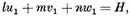(c) through the interior,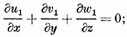u1, v1, w1 may be taken as any three quantities whatever for which these equations hold.

Let Q be the actual vis-viva of the liquid, and Q1 the vis-viva it would have if u1, v1, w1 represented its motion. We shall have, calling ρ the density,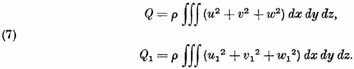From these we deduce, by subtraction and an algebraic modification,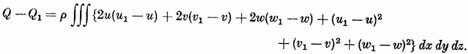Now, according to the proposition proved by Mr. Stokes in Note V, since the fluid was initially ["primitively"] at rest,2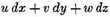must be the differential of some function of x, y, z (involving also, in general, t as another independent variable), which we may denote by φ; so that we have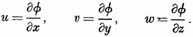Hence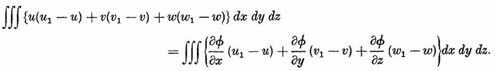Integrating the first term by parts with respect ["reference"] to x, the second with respect to y, and the third with respect to z, we reduce the second member to the formThe triple integral here vanishes in virtue of equations (b) and (c); and the double integral, which is to be extended over the entire bounding surface, may3 be put under the form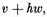This also vanishes in virtue of equations (4) and (b);4 and hence the definite integral under consideration vanishes. Thus we see that the expression for Q1Q reduces to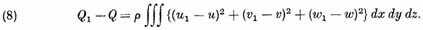In this expression the factor of dx dy dz, and consequently the entire integral, is essentially positive, unless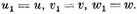Hence, of all the states of motion of the fluid kinematically possible at each instant, any one which differs from the actual state of motion possesses a greater vis-viva. Q.E.D.

6. COR. 1. The conditon that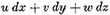must be a complete differential is, in addition to the kinematical relations, sufficient to determine the motion. For in the preceding demonstration no other condition was introduced to characterize u. v, w. 5

COR. 2. The motion of the fluid at any time is independent of the preceding motion, and depends solely on the given form and normal motion of the bounding surface at the instant.

COR. 3. If the bounding surface, after having been in motion, be brought to rest in any position, the liquid will, at the same instant, be reduced to rest.

7. The expression for the vis-viva of the liquid may be put into a very remarkable form, by making use of the differential coefficients of φ in place of u, v, and w, and then integrating by parts in the following manner. Thus we have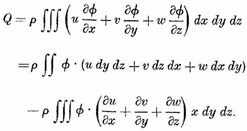The triple integral vanishes in virtue of equation (5), and the double integral, extended over the bounding surface, may be modified by the transformation employed in §4, so that we have the following expression for the vis-viva,6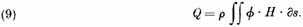The variation of the function φ within the bounding surface will not affect the value of this integral, in which φ may be considered as merely a function of the coordinates of a point in the surface itself. Hence, while the factor H expresses the given normal velocities at the different points of the containing surface, the other factor, φ, under the integral sign, is such as to express by its differential components with reference to superficial coordinates, the tangential component of the velocity of a particle of the fluid in contact with this surface.

1 W. Thomson Lord Kelvin "Notes on hydrodynamics. On the vis-viva of a liquid in motion," Cambridge Dublin Math. J. 4 (1849), 90; Collected Papers, pp. 107–112.

2 This is the proposition (due to Lagrange and Cauchy) that initially irrotational motion remains irrotational. See Lamb’s Hydrodynamics (Cambridge University Press, Cambridge, England, 6th ed., 1932), p. 12, for further references.

3 The editor refers the reader to Note I, vol. ii, p. 285, of Kelvin’s Collected Papers.

4 Equation (4) states that the normal velocity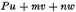is a fixed function H of position.

5 The editor refers the reader to vol. III, p. 86, where similar reasoning was applied to prove a theorem, of which the corollary in the text is a particular case.

6 This is a particular case of a general theorem proved in an article entitled "Propositions in the Theory of Attraction, Part II," being obtained by taking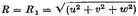and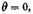in equations (3) and (4) of that article (Old Series, vol. III, p. 202. (W. T.)

Contents:

William Thomson

## Download Options

Title: A Source Book in Classical Analysis

Select an option:

*Note: A download may not start for up to 60 seconds.

## Email Options

Title: A Source Book in Classical Analysis

Select an option:

Email addres:

*Note: It may take up to 60 seconds for for the email to be generated.

Chicago: William Thomson et al., "70b. Kelvin on a Variational Principle of Hydrodynamics," A Source Book in Classical Analysis in A Source Book in Classical Analysis, ed. Garrett Birkhoff (Cambridge: Harvard University Press, 1973), 383–385. Original Sources, accessed April 18, 2021, http://www.originalsources.com/Document.aspx?DocID=442PWG9RLVEZ6GI.

MLA: Joule, James Prescott, et al. "70b. Kelvin on a Variational Principle of Hydrodynamics." A Source Book in Classical Analysis, Vol. 4, in A Source Book in Classical Analysis, edited by Garrett Birkhoff, Cambridge, Harvard University Press, 1973, pp. 383–385. Original Sources. 18 Apr. 2021. www.originalsources.com/Document.aspx?DocID=442PWG9RLVEZ6GI.

Harvard: Joule, JP et al., '70b. Kelvin on a Variational Principle of Hydrodynamics' in A Source Book in Classical Analysis. cited in 1973, A Source Book in Classical Analysis, ed. , Harvard University Press, Cambridge, pp.383–385. Original Sources, retrieved 18 April 2021, from http://www.originalsources.com/Document.aspx?DocID=442PWG9RLVEZ6GI.